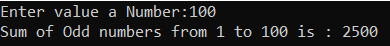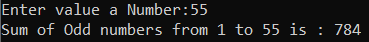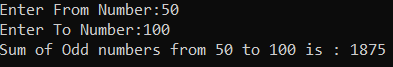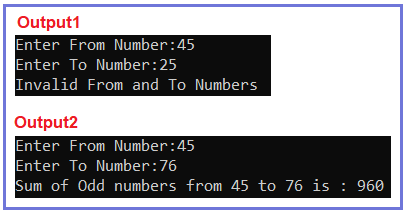# Find sum of odd numbers from 1 to N in C#

## How to Find the sum of odd numbers from 1 to N in C# with Examples

In this article, I am going to discuss How to find the sum of odd numbers from 1 to N in C# with Examples. Please read our previous article, where we discussed How to find the sum of even numbers from 1 to N in C#. Here, in this article, first, we will discuss what are Odd Numbers, then we will discuss how to find the sum of odd numbers between a range of numbers and finally, we will see how to implement the sum of odd number Program in C#.

###### What are Odd Numbers?

An odd number is a number that is not divisible by 2. The remainder in the case of an odd number is always 1. Example: 1, 3, 5, 7, 9, etc.

###### How to check if a number is odd or not?

If a number is not divisible by 2 then it is an odd number.
if(number % 2 == 1)
This is an odd number.
else
This is not an odd number.

##### How to Print the sum of odd numbers from 1 to N in C#?

In the case of numbers from 1 to 100, there are 50 odd numbers and 50 even numbers. This basically means every second number is an odd number.
Example: 1, 3, 5, 7, 9, etc. are odd numbers
1 is an odd number
then 3 (2 is an even number)
then 5 (4 is an even number)
5 then 7 (6 is an even number) and so on.

##### Algorithm to find the sum of odd numbers

Step1: Take a variable named N and store the value of the upper limit of N.
Step2: Take one more variable named sum to store the result of the calculation.
Step3: Take a for loop with variable i which be initialized with 1 and in condition expression check for (i<=N) and
Step4: In the third expression of for loop increment the value of i with 2(because every second number is odd/even).
for(int i=1;i<=N;i=i+2)
sum += i; //sum = sum + i;

##### Example: C# Program to find the sum of odd numbers from 1 to n

The following C# Program will find the sum of odd numbers from 1 to N using for loop.

```using System;
namespace DotNetTutorials
{
class SumOfEvenNumbers
{
static void Main(string[] args)
{
int sum = 0;
Console.Write("Enter value a Number:");

//1+2 = 3
//3+2 = 5
for (int i = 1; i <= Number; i += 2)
{
sum += i;
}
Console.Write(\$"Sum of Odd numbers from 1 to {Number} is : {sum}");
}
}
}
```
###### Output:##### Example: Using while loop

The following C# Program will find the sum of odd numbers from 1 to N using the while loop.

```using System;
namespace DotNetTutorials
{
class SumOfEvenNumbers
{
static void Main(string[] args)
{
int sum = 0, i = 1;
Console.Write("Enter value a Number:");

while (i <= Number)
{
sum += i;
i += 2;
}

Console.Write(\$"Sum of Odd numbers from 1 to {Number} is : {sum}");
}
}
}
```
###### Output:##### Finding the sum of odd numbers between a Range of Numbers:

The examples we have discussed earlier are used to find the sum of odd numbers starting from 1. But what if we don’t want to start from 1, instead we can give any numbers, let’s say we want to find the sum of odd numbers from 50 to 100. The following C# program will ask the user to enter two numbers (the first number should be less than the second number) and then calculate the sum of odd numbers.

```using System;
namespace DotNetTutorials
{
class SumOfEvenNumbers
{
static void Main(string[] args)
{
int sum = 0;
Console.Write("Enter From Number:");

Console.Write("Enter To Number:");

if (FromNumber < ToNumber)
{
for (int i = FromNumber; i <= ToNumber; i++)
{
if (i % 2 == 1)
sum += i;
}

Console.Write(\$"Sum of Odd numbers from {FromNumber} to {ToNumber} is : {sum}");
}
else
{
Console.Write("Invalid From and To Numbers");
}

}
}
}
```
###### Output:##### Using Function

In the below C# program, we have created one method to find the sum of odd numbers between a range of numbers.

```using System;
namespace DotNetTutorials
{
class SumOfEvenNumbers
{
static void Main(string[] args)
{
int sum = 0;
Console.Write("Enter From Number:");

Console.Write("Enter To Number:");

if (FromNumber < ToNumber)
{
sum = FindSumOfOddNumbers(sum, FromNumber, ToNumber);
Console.Write(\$"Sum of Odd numbers from {FromNumber} to {ToNumber} is : {sum}");
}
else
{
Console.Write("Invalid From and To Numbers");
}

}

private static int FindSumOfOddNumbers(int sum, int FromNumber, int ToNumber)
{
for (int i = FromNumber; i <= ToNumber; i++)
{
if (i % 2 == 1)
sum += i;
}
return sum;
}
}
}
```
###### Output:In the next article, I am going to discuss How to Convert a Kilogram to Gram and vice versa in C# with Examples. Here, in this article, I try to explain How to Find the sum of odd numbers from 1 to N in C# with Examples and I hope you enjoy this How to Find the sum of odd numbers from 1 to N article.